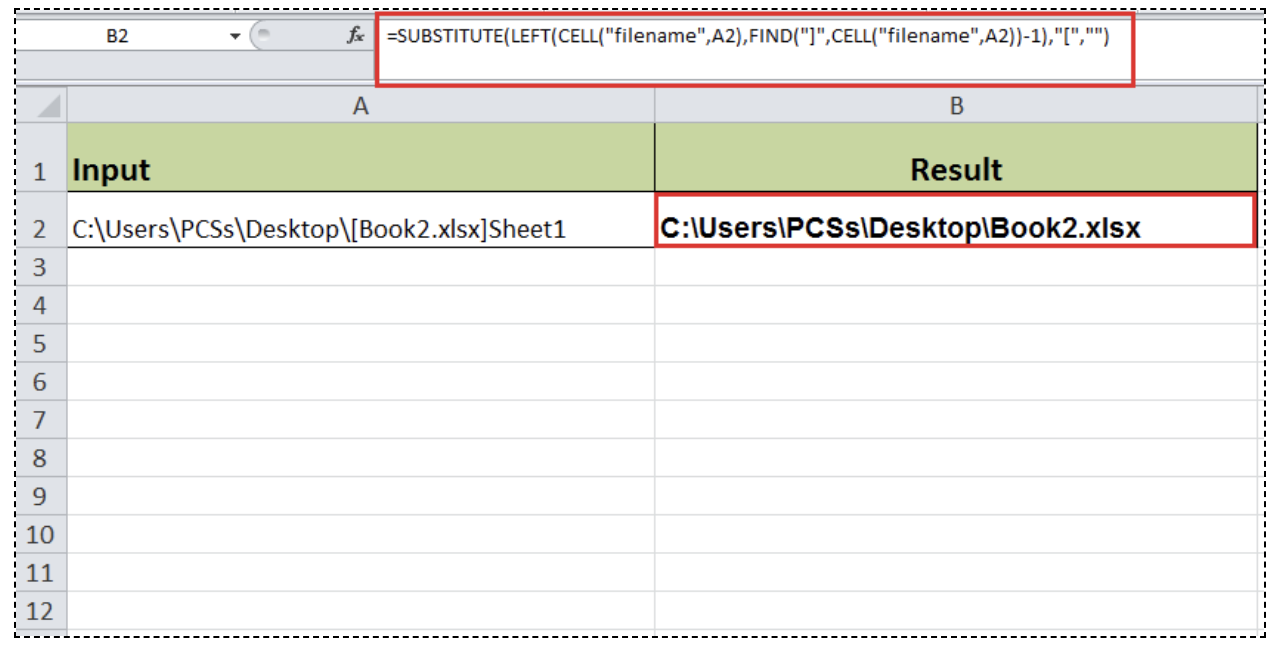Get instant live expert help with Excel or Google Sheets“My Excelchat expert helped me in less than 20 minutes, saving me what would have been 5 hours of work!”

#### Post your problem and you'll get expert help in seconds

Your message must be at least 40 characters
Our professional experts are available now. Your privacy is guaranteed.

# How to Get a Workbook Name and Path without Sheet in Excel

Is it accurate to say that you are hoping to get workbook name and path without making use of the sheet name? You can achieve this by using a formula based on various Excel functions like SUBSTITUTE, LEFT, FIND and CELL functions to return the final result.

## Get a workbook name and path without sheet name in Excel

With the combined functions mentioned above, you can find the workbook’s name and path without sheet name, that looks like this:

Path\Workbook_name.xlsx

### Formula

`=SUBSTITUTE(LEFT(CELL("filename",A1),FIND("]",CELL("filename",A1))-1),"[","")`

### Explanation

This formula is a combination of Information and String/Text functions. The following step-by-step explanation will present the formula as below:

• The CELL function using the “filename” option returns the name and path of the full workbook and this part of the formula:

CELL(“filename”,A1)

It returns the result as follows:

path\[Workbook_name.xlsx]Sheetname

• The above full workbook path is then taken into the LEFT function as a text string, and following FIND function returns the position of closing square bracket “]”, minus 1:

FIND(“]”,CELL(“filename”,A1))-1

The LEFT function returns the following result after extracting the text string:

path\[Workbook_name.xlsx

• The above text string is then received in SUBSTITUTE function to replace opening square bracket “[“ with a blank string.

This is how it looks like:

`=SUBSTITUTE(“path\[workbook_name.xlsx”,"[","")`

### Example

Secondly, we will go through an example as Figure 1. Suppose we have a workbook’s full name, along with its complete path and sheet name in cell A2. We want to get only workbook’s name and path, without a sheet name. Let’s have a look at following image where we will get workbook name and path without sheet name as shown by using the following formula in the cell:

`=SUBSTITUTE(LEFT(CELL("filename",A2),FIND("]",CELL("filename",A2))-1),"[","")`

As a result, this formula will return:

path\Workbook_name.xlsxFigure 1. Get workbook name and path without sheet

Still need some help with Excel formatting or have other questions about Excel? Connect with a live Excel expert here for some 1 on 1 help. Your first session is always free.

### Did this post not answer your question? Get a solution from connecting with the expert.Another blog reader asked this question today on Excelchat:## Subscribe to Excelchat.coAnother blog reader asked this question today on Excelchat: